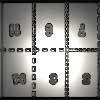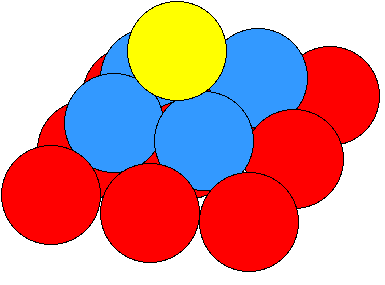You may also likeExploring Wild & Wonderful Number Patterns

EWWNP means Exploring Wild and Wonderful Number Patterns Created by Yourself! Investigate what happens if we create number patterns using some simple rules.Magazines

Let's suppose that you are going to have a magazine which has 16 pages of A5 size. Can you find some different ways to make these pages? Investigate the pattern for each if you number the pages.Pebbles

Place four pebbles on the sand in the form of a square. Keep adding as few pebbles as necessary to double the area. How many extra pebbles are added each time?

Pyramid Numbers

Age 7 to 11Challenge Level

Thank you to Adrian from South Greenhoe Middle School, Lucy and Sarah from Archbishop Sancroft High School and pupils from Heacham Middle School who all sent correct solutions. Here is a summary of their responses:

The sequence is generated by adding successive square numbers
$1 = 1^2$
$5 = 1^2 + 2^2$
$14 = 1^2 + 2^2 + 3^2$

The next three numbers in the sequence will be
$1^2 + 2^2 + 3^2 + 4^2 = 30$
$1^2 + 2^2 + 3^2 + 4^2 + 5^2 = 55$
$1^2 + 2^2 + 3^2 + 4^2 + 5^2 + 6^2 = 91$

The numbers are called pyramid numbers because if you take some balls of the same size and you build them into pyramids, you will need to make square-based pyramids with one ball at the top, four in the next layer down, nine in the next layer down and so on.Similar sequences to this are triangular numbers (1, 3, 6, 10 .... ), which are sums of whole numbers, and (1, 9, 36, 100,... ) which are the sums of cubes.

Perhaps you can draw pictures for these sequences too?